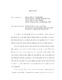## Mean field limit for stochastic particle systems with singular forcesIn this thesis, we systematically study the mean field limit for large systems of particles interacting through rough or singular kernels by developing a new statistical framework, based on controlling the relative entropy between the $N-$particle distribution and the limit law through identifying new Laws of Large Numbers. We study both the canonical 2nd order Newton dynamics and the 1st order (kinematic) systems, leading to McKean-Vlasov systems in the large $N$ limit. For the 2nd order case, we only require that the interactions $K$ be bounded. The control of the relative entropy implies the mean field limit and the propagation of chaos through the strong convergence of all the marginals. For the 1st order case, with the help from noise we can even obtain the mean field limit for interactions $K \in W^{-1, \infty}$, {\em i.e.} the anti-derivatives of $K$ are bounded (or even unbounded with weak singularity). To our knowledge, this is the first time the relative entropy method applied to obtain the mean field limit. Compared to the classical framework with $K \in W^{1, \infty}$, our results show another critical scale $K \in L^\infty$ for the mean field limit. Our results are quantitative: we can provide precise control of the relative entropy and hence the convergence of the marginals. We expect that the relative entropy method will be another standard tool in the study of the mean field limit. This thesis resulted in the publications \cite{JW1, JW2, JW3}.